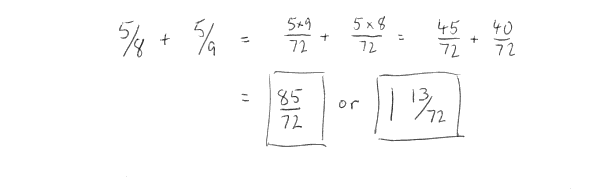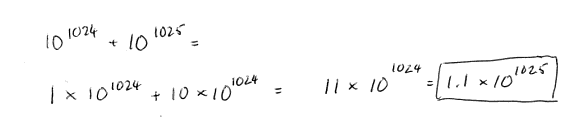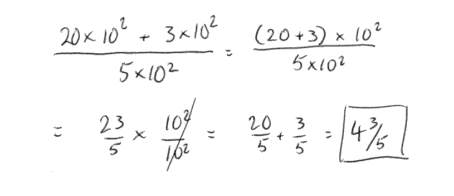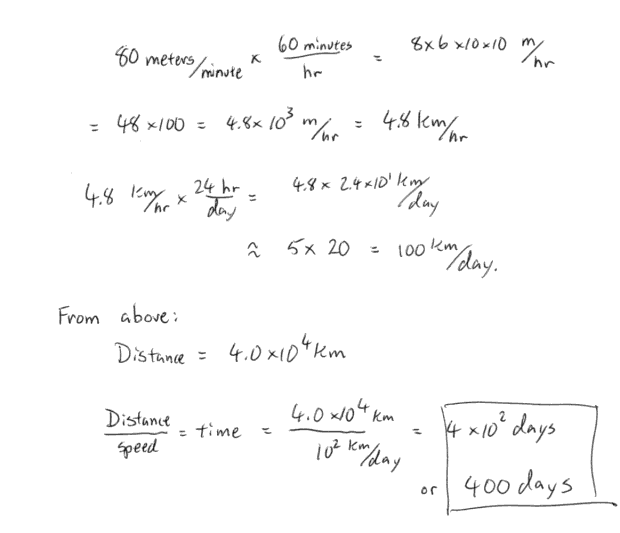Back to assignments

A100 -- Dan Swearingen
September 3, 1996: Basic Math Skills

Copy and work out problems on you own paper. Turn in before you leave tonight. No calculators allowed.

Solve, simplify, and/or reduce the following problems:

1. Five-eighths added to five ninths2. Write 87,655,342,190,890.9865 in scientific notation.

8.76553421908909865 x 1013

3. Write out "One hundred eighty million" in scientific notation.

180,000,000. = 1.8 x 108

4. 101024 + 101025 =5. 101024 / 101025 =6.=For the following problems it is acceptable to round numbers off to make solving the problem easier. For example pi = 3.1415926... or about 3. Using 3 for pi is fine (as long as you aren=t designing an airplane!).

7a. If the diameter of the Earth is 12,756 km, how many km would you have to walk if you walked around the Earth once? The area of a circle is pi x R2, where R is the radius. The circumference of a circle is 2pi x R.7b. If you walk at a speed of 80 meters per minute, how many days would it take for you to walk around the Earth once (assuming you're truly special and can walk on water)?Back to assignments